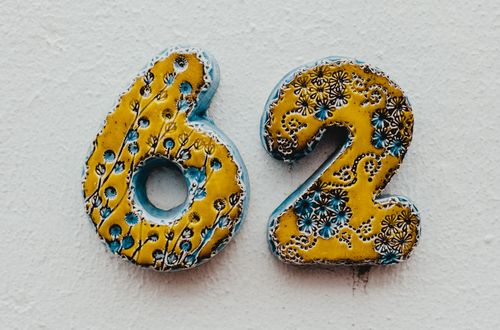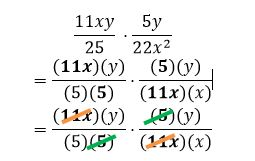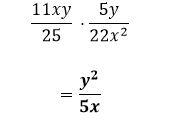# How to multiply algebraic fractions

Want to find out how to multiply algebraic fractions? We've provided a step-by-step guide of how to multiply algebraic fractions to teach you everything you need to know.## Step 1: Cancel out common factors

Start by cancelling out the common factors shared by the numerator and the denominator. For example if we want to multiply 3a/(5b^2 ) and (25b^3)/(27a^4 ).

## Step 2: Multiply the remaining factors

Find the product my multiplying the remaining factors:

## Examples of how to multiply algebraic fractions

Q1) What is the product of 11xy/25 and 5y/(22x^3 )?

Look for the common factors shared by the numerator and denominator and cancel out common factors.Multiply the remaining factors to find the product.#### Join today

The fastest way to practice

Unlock our complete testing platform and improve faster that ever.